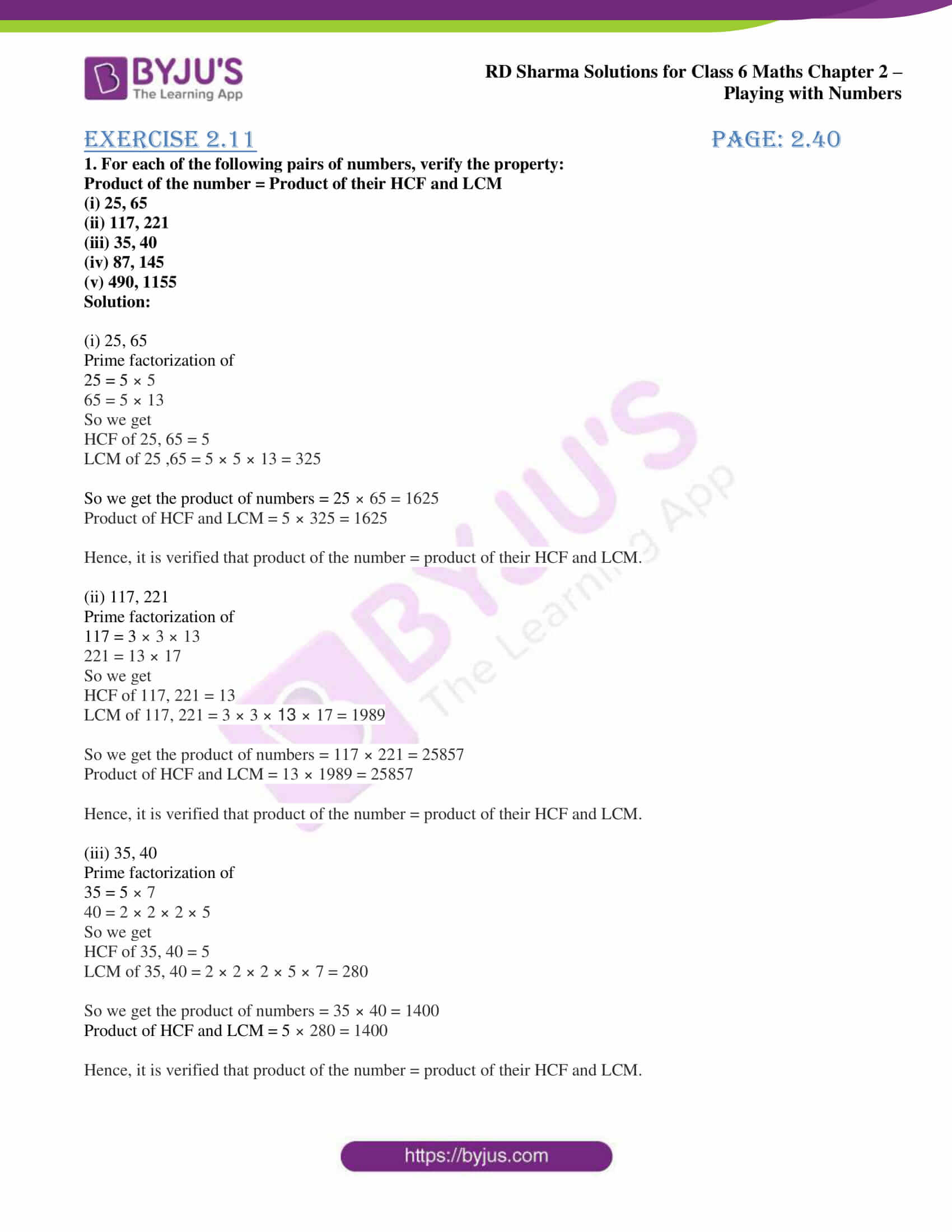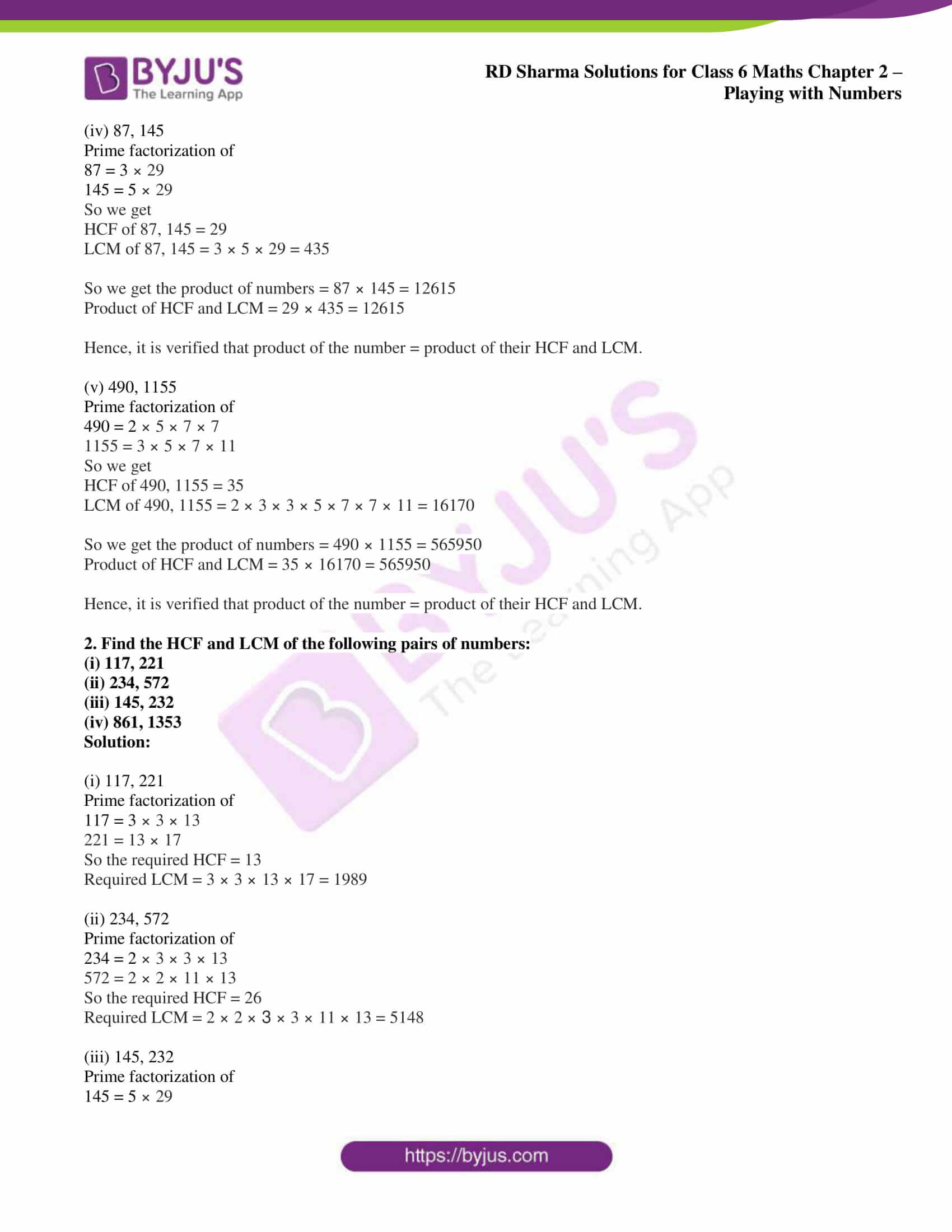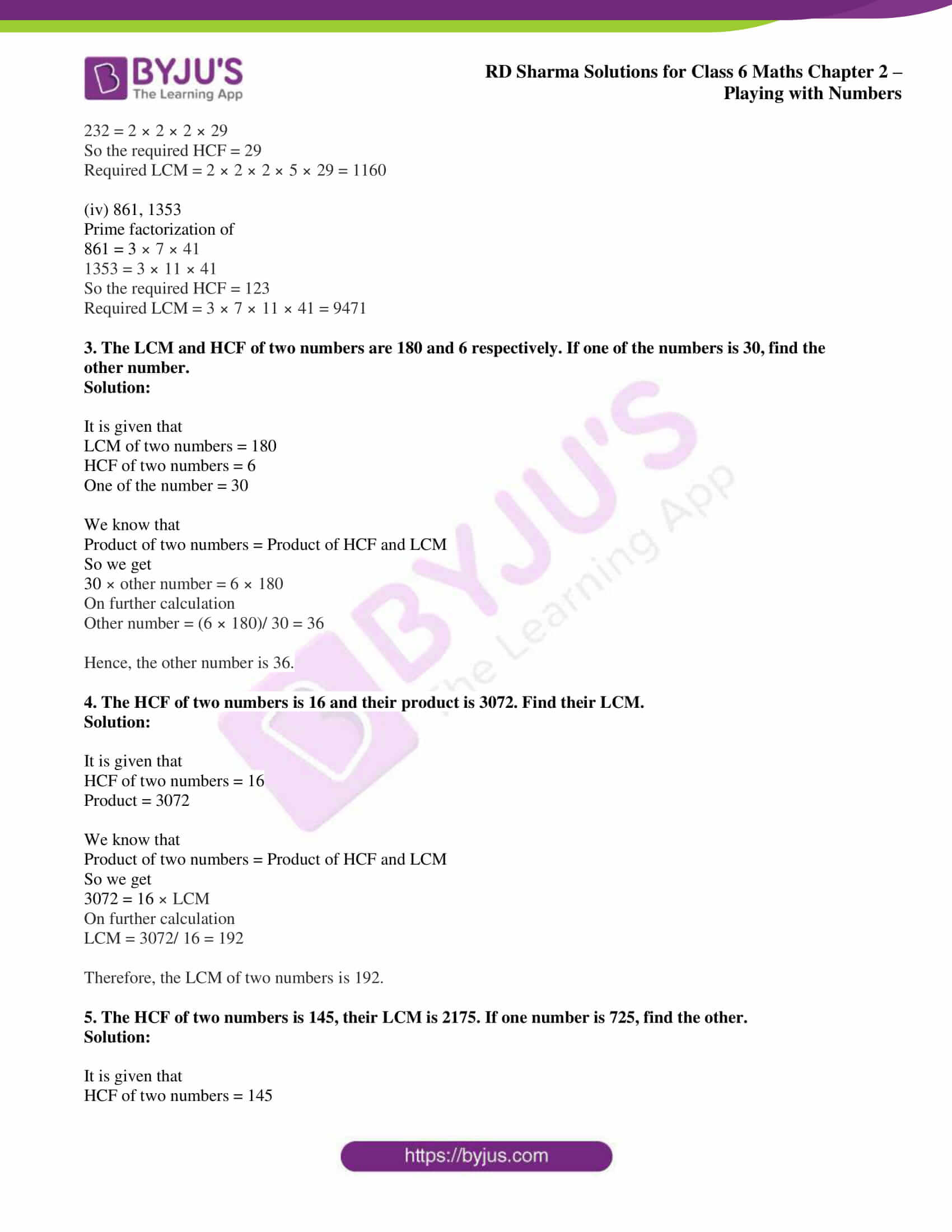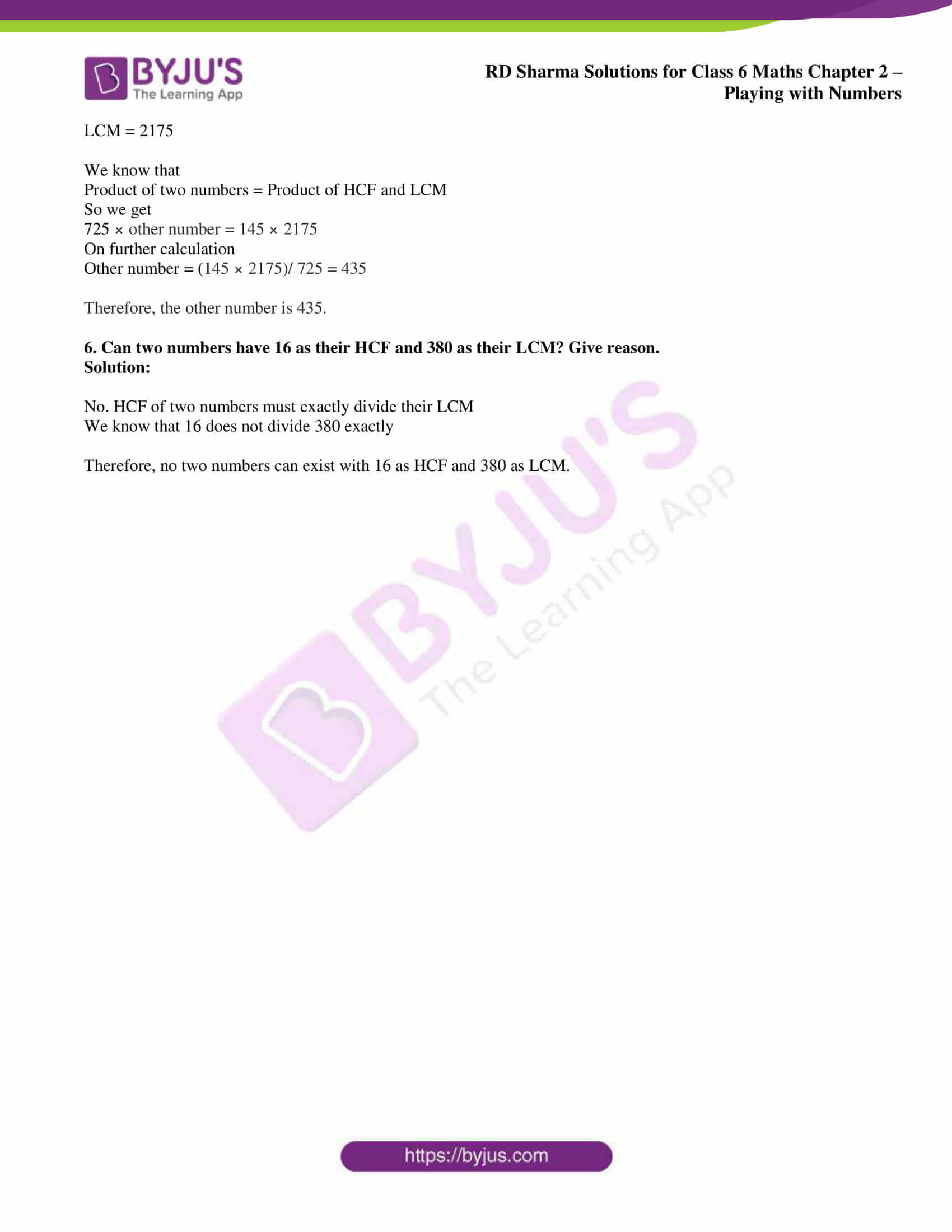# RD Sharma Solutions for Class 6 Maths Chapter 2: Playing with Numbers Exercise 2.11

## RD Sharma Solutions for Class 6 Maths Exercise 2.11 PDF

Each concept is explained in a comprehensive manner with huge number of examples to make them easier for the students. The solutions can be used as a reference material by the students which helps in performing well in the exam for Class 6. The students can self analyse their performance and spend more time on their areas of weaknesses. The solutions of exercise wise problems are available in PDF format, which can be downloaded for free by the students. RD Sharma Solutions for Class 6 Maths Chapter 2 Playing with Numbers Exercise 2.11 are provided here.

## RD Sharma Solutions for Class 6 Maths Chapter 2: Playing with Numbers Exercise 2.11 Download PDF## Access answers to Maths RD Sharma Solutions for Class 6 Chapter 2: Playing with Numbers Exercise 2.11

1. For each of the following pairs of numbers, verify the property:

Product of the number = Product of their HCF and LCM

(i) 25, 65

(ii) 117, 221

(iii) 35, 40

(iv) 87, 145

(v) 490, 1155

Solution:

(i) 25, 65

Prime factorization of

25 = 5 × 5

65 = 5 × 13

So we get

HCF of 25, 65 = 5

LCM of 25 ,65 = 5 × 5 × 13 = 325

So we get the product of numbers = 25 × 65 = 1625

Product of HCF and LCM = 5 × 325 = 1625

Hence, it is verified that product of the number = product of their HCF and LCM.

(ii) 117, 221

Prime factorization of

117 = 3 × 3 × 13

221 = 13 × 17

So we get

HCF of 117, 221 = 13

LCM of 117, 221 = 3 × 3 × 13 × 17 = 1989

So we get the product of numbers = 117 × 221 = 25857

Product of HCF and LCM = 13 × 1989 = 25857

Hence, it is verified that product of the number = product of their HCF and LCM.

(iii) 35, 40

Prime factorization of

35 = 5 × 7

40 = 2 × 2 × 2 × 5

So we get

HCF of 35, 40 = 5

LCM of 35, 40 = 2 × 2 × 2 × 5 × 7 = 280

So we get the product of numbers = 35 × 40 = 1400

Product of HCF and LCM = 5 × 280 = 1400

Hence, it is verified that product of the number = product of their HCF and LCM.

(iv) 87, 145

Prime factorization of

87 = 3 × 29

145 = 5 × 29

So we get

HCF of 87, 145 = 29

LCM of 87, 145 = 3 × 5 × 29 = 435

So we get the product of numbers = 87 × 145 = 12615

Product of HCF and LCM = 29 × 435 = 12615

Hence, it is verified that product of the number = product of their HCF and LCM.

(v) 490, 1155

Prime factorization of

490 = 2 × 5 × 7 × 7

1155 = 3 × 5 × 7 × 11

So we get

HCF of 490, 1155 = 35

LCM of 490, 1155 = 2 × 3 × 3 × 5 × 7 × 7 × 11 = 16170

So we get the product of numbers = 490 × 1155 = 565950

Product of HCF and LCM = 35 × 16170 = 565950

Hence, it is verified that product of the number = product of their HCF and LCM.

2. Find the HCF and LCM of the following pairs of numbers:

(i) 117, 221

(ii) 234, 572

(iii) 145, 232

(iv) 861, 1353

Solution:

(i) 117, 221

Prime factorization of

117 = 3 × 3 × 13

221 = 13 × 17

So the required HCF = 13

Required LCM = 3 × 3 × 13 × 17 = 1989

(ii) 234, 572

Prime factorization of

234 = 2 × 3 × 3 × 13

572 = 2 × 2 × 11 × 13

So the required HCF = 26

Required LCM = 2 × 2 × 3 × 3 × 11 × 13 = 5148

(iii) 145, 232

Prime factorization of

145 = 5 × 29

232 = 2 × 2 × 2 × 29

So the required HCF = 29

Required LCM = 2 × 2 × 2 × 5 × 29 = 1160

(iv) 861, 1353

Prime factorization of

861 = 3 × 7 × 41

1353 = 3 × 11 × 41

So the required HCF = 123

Required LCM = 3 × 7 × 11 × 41 = 9471

3. The LCM and HCF of two numbers are 180 and 6 respectively. If one of the numbers is 30, find the other number.

Solution:

It is given that

LCM of two numbers = 180

HCF of two numbers = 6

One of the number = 30

We know that

Product of two numbers = Product of HCF and LCM

So we get

30 × other number = 6 × 180

On further calculation

Other number = (6 × 180)/ 30 = 36

Hence, the other number is 36.

4. The HCF of two numbers is 16 and their product is 3072. Find their LCM.

Solution:

It is given that

HCF of two numbers = 16

Product = 3072

We know that

Product of two numbers = Product of HCF and LCM

So we get

3072 = 16 × LCM

On further calculation

LCM = 3072/ 16 = 192

Therefore, the LCM of two numbers is 192.

5. The HCF of two numbers is 145, their LCM is 2175. If one number is 725, find the other.

Solution:

It is given that

HCF of two numbers = 145

LCM = 2175

We know that

Product of two numbers = Product of HCF and LCM

So we get

725 × other number = 145 × 2175

On further calculation

Other number = (145 × 2175)/ 725 = 435

Therefore, the other number is 435.

6. Can two numbers have 16 as their HCF and 380 as their LCM? Give reason.

Solution:

No. HCF of two numbers must exactly divide their LCM

We know that 16 does not divide 380 exactly

Therefore, no two numbers can exist with 16 as HCF and 380 as LCM.

### RD Sharma Solutions for Class 6 Maths Chapter 2 – Playing with Numbers Exercise 2.11

RD Sharma Solutions Class 6 Maths Chapter 2 Playing with Numbers Exercise 2.11 explains the important properties of HCF and LCM of given numbers.

### Key features of RD Sharma Solutions for Class 6 Maths Chapter 2: Playing with Numbers Exercise 2.11

• The solutions created by subject experts help in obtaining overall idea about the concepts.
• Self study method is improved among students which helps them clear doubts by themselves referring to the PDF.
• Important formulas, shortcuts and tips are highlighted in order to help students grasp the concepts faster.
• Huge number of examples before each exercise improves knowledge among students about the various methods used in solving problems.